Email Us: info@lupinepublishers.comCall Us: +1 (914) 407-6109   57 West 57th Street, 3rd floor, New York - NY 10019, USA

# Lupine Publishers Group

## Lupine Publishers

Submit Manuscript

ISSN: 2643-6744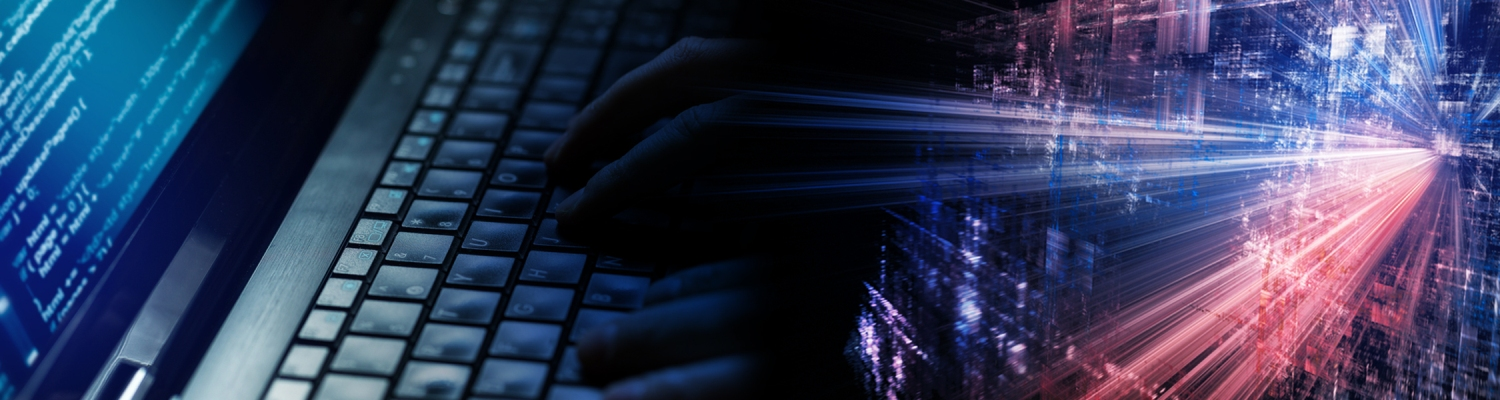# Quantum Intelligence Volume 1 - Issue 4

Chi Tran*

• Department of Computer Science, WM University, Poland

Received:August 28, 2019;   Published: September 13, 2019

*Corresponding author: Chi Tran, Department of Computer Science, WM University, Poland.

DOI: 10.32474/CTCSA.2019.01.000118

## Abstract

On the one hand, we recognize the real world using usually intuitive perceptions through physical experiments and intuitive perception processing based on binary philosophy. However, physical quantum phenomenon indicates that each photon transfers in the way not agreement with the soul of binary philosophy. That is to say that we cannot use intuitive perception based on binary philosophy to understand the quantum world of subatomic transmitted with the light speed. On the other hand, the simultaneous appearance of two intuitive perceptions: ‘being’ and ‘non-being’ at the same time in the human brain, according to Georg Wilhelm Friedrich Hegel (1770-1831), created a counterintuitive perception expressed by rather meta language, ‘being-nonbeing’ that goes beyond the boundaries of the description of a common language. It is seen as the quantum perception and the quantum cognitive phenomenon originated from activity of the human neural network, used to qualify/quantify the changes of environmental conditions and to increase the potential of the human brain. In addition, Hegel noted that the reality was saturated in the “Ones”, we do usually split up and rearrange it. These Hegel’s ideas are considered as the quantum philosophy (the ‘One’ philosophy) and related logic called quantum logic – the human philosophy and logic rather than binary philosophy and two valued logic used in computer. It creates the main foundation of real-time intelligence in the so, called dynamic quantum dimension of the human brain. Today, scientists of artificial intelligence are aware that they consider the real world through the human brain, the virtual world of the physical world, i.e. They explore the real world in a virtual world only. As, the human brain is really a physical world, we explore the real world through another physical world. Information in the human brain is like the subatomic in the physical quantum world, communicates with the speed of light creating a world called the quantum cognitive world. It indicates that we should use the characteristic homology of quantum perception processing and quantum bit, qubit, processing to not only better exploit the power and efficiency of the human brain but also to build a new architecture of the so called the quantum cognitive computer in the future. In this paper, we want to simulate an ability of the human brain by a multimodal approach to comprehensively and more accurately understand how the human brain can quantify the rapid change of environmental conditions in real time. The equality of the quantum perception processing and quantum bit processing enables us to create a new approach of embedded software design and a new platform of IoT technology, new model of machine learning and new field of cognitive robotics, etc., which results in a new model of artificial intelligence named Quantum Intelligence.

Keywords: Quantum perception; Quantum Philosophy; Dynamic Quantum Dimension; Quantum Perception Processing; Quantum Bit Processing; Quantum Intelligence

## Introduction

On the one hand, the quantum physical phenomenon is discovered from the physical experiment carried out by Thomas Young (1801) that indicates that, each photon of light, passes through both slits at the same time. On the other hand, the simultaneous appearance of two intuitive perceptions, ‘being’ and ‘nonbeing’ in the human brain, confirmed by Georg Wilhelm Friedrich Hegel (1770-1831) creates a new counter-intuitive perception expressed by the meta-language, ‘being-nonbeing’ with content beyond our understanding by common language. In addition, Hegel noted that reality was saturated in “One” and we often split and rearrange it. These Hegel’s ideas express the so called the quantum philosophy, which is seen as the base of the human thought – the human philosophy, not binary philosophy used in computer. The human brain is in fact the virtual world of the real world. It is a physical world, in which information transmitted at the speed of light, see , has created the so-called quantum cognitive world. Unseparated perception, ‘truefalse’ is, for example, considered as the quantum perception of truth, truth comes from the future, [2-4] or the human truth described in the multi-valued logic of [5-7] which is fundamental of the so called quantum logic rather than two valued logic. In another way, the denotation concept introduced by , according to the author understands, expressed the human thought described by logical values rather than common words. We should use the ideas of above authors, to create the so-called dynamic quantum cognitive dimension that demonstrates another capability of the human brain to recognize the physical world in real time. While functioning of quantum physical phenomenon is based on the quantum physics, the functioning of quantum cognitive phenomena is based on quantum philosophy and quantum logic. We should change the way of thinking to better exploit the power and efficiency of Human brain in order to build a new model of real-time intelligence called Quantum Intelligence, QI, and to build a new architecture of the so-called quantum cognitive computer in the future. The same base of activity of the human brain and the quantum cognitive computer enables us to create a new approach of embedded software design and a new platform of IoT technology, new model of machine learning and new field of cognitive robotics, etc.

## Real Time Intelligence

We recognize the real world through the sense organ such as seeing, hearing and others. For example, when eyes and ears receive the danger signals from the front of the boy, he must decide immediately using the available experience and results of real-time information processing. This decision is transmitted at once to the legs (actuator) to run in the right direction. The danger is increasing from the constant changes of fire and environmental conditions, transmitted in a stream form. He must quickly model the actual dangerous state through an ability of qualification/quantification of the fire speed, wind speed, etc. and making quick decisions depending entirely on cognitive ability about the urgent reality that leads to the boy’s immediate action - real time intelligence. That is: (Figure 1).

## Quantum Perception

Perception is defined by Akṣapāda Gautama in his Nyaya Sutra (I,i.4) as a 'non-erroneous’ cognition, which is produced by the intercourse of sense-organs linked to the human brain with the real objects. It includes intuitive perception, Laukika, and counterintuitive perceptions, Alaukika. While, Laukika rationalizes the level of objectivity and confines under the syntactic constraints; Alaukika rationalizes a new level of objectivity under semantics and pragmatics aspects that are beyond our understanding by Laukika. The appearance of two intuitive perceptions, ‘Being’ and ‘Nonbeing’ at the same time, according to Georg Wilhelm Friedrich Hegel (1770-1831), creates a new counterintuitive perception: 'Being-Nonbeing' which we call quantum perception. In fact, Hegel confirmed that the reality was saturated in the 'One' and we usually subdivide and rearrange it. Hegel's idea is considered a quantum philosophy (the "One" philosophy, see . It is shown in Figure 2. This quantum philosophy is derived from the actions of the human brain's neural network to quickly adapt to changes in real environmental conditions in real time. While in the physical quantum world, subatomic being moved at the speed of light, in the human brain, information is moved with the speed of light also. It creates a special ability of the human brain to flexibly recognize changes of the real world in real time i.e., real time intelligence which we call the Quantum Intelligence, QI. In another way,  performs one of new science principles of cognition. Author has developed a theory of sense and denotation into thoroughgoing philosophy of language. It is developed from the information processing approach toward psychology as an attempt to develop a new cognitive trend that mimics the human's capacity to use knowledge in an intelligent way. By the term denotation understood as the hidden thought of the human brain, which should be a quantum cognitive measurement, t, that goes beyond what we can understand by ordinary language. It can be expressed by:

‘Being-Nonbeing’ = ‘Being-Nonbeing’|thought = Being-Nonbeing|τ(Being) ˄Being-Nonbeing τ (Nonbeing) (1)
Subject to
τ (Being) + τ (Nonbeing) = 1

## Quantum Perception Processing

In a traditional computer, reasoning with two separated intuitive states: ‘Being’ (B) and ‘Nonbeing’ (N) is represented by:

‘Being’ or ‘Nonbeing’ = {B,
ØN} = 21 intuitive possibilities (2)
While, in the quantum cognitive world, each quantum perception exists in more than one state simultaneously: ‘Being’ presents by two states (B, ¬B) and ‘Non-being’ by (N, ØN), then processing of quantum perception, ‘Being-Nonbeing’, is presented in the form:
‘Being-Nonbeing’ = BN, ΒØΝ,
¬BΝ, ¬ΒØN} = 22 quantum possibilities (3)
In this case, it results in exponential increase (22) of quantum possibilities.

Similarly, using four-cornered argumentation of the 4-valued cognitive logic (Indian logic), in which we have: the box connective, ■ (affirmation), and diamond connective, ♦(both ■ and ¬■), for the four-cornered argumentation: ■, ♦, ¬■ and ¬♦; we can obtain exponential growth 24 rather than 22 quantum possibilities. That is:

‘Being-Nonbeing’ = {■B■N, ■B♦N, ■B¬■N, ■B¬♦N,
♦B■N,♦B♦N,♦B¬■N,♦B¬♦N,
¬■B■N, ¬■B♦N, ¬■B¬■N, ¬■B¬♦N,
¬♦B■N, ¬♦B♦N, Ø♦B¬■N, ¬♦

B¬♦N} = 24 (4)

As, Indian 4-valued cognitive logic is a restriction of Łukasiewicz’s n-valued cognitive logic with n = 4. By the same way, we have:

i. n = 2 ⇒ exponential growth 22
ii. n = 3 ⇒ exponential growth 23
iii. n = 4 ⇒ exponential growth 24
iv. n = n ⇒ exponential growth 2n

It indicates really that quantum perception processing using quantum logic (multi-valued logic) is the key to increase exponentially (n-degree growth) of the human brain potential.   Quantum intelligence is seen as real time intelligence of the human brain based on the so-called dynamic quantum truth dimension. It is presented as:

‘true-false’ = ‘true-false’|thought = {(‘true-false’|τ(true)) ∧ (‘true-false’|τ(false))} (5)
Subject to
τ(true) + τ(false) = 1

in which, t is considered as quantum cognitive measurement; Ù denotes the logical operator (AND); the human thought is characterized by quantum cognitive measurement t, see [9-12].

As, relative changing between τ (true) and τ(false) expresses an ability to easily change the people’s thinking that ensures the flexible changing dynamically of the quantum cognitive measurements. It presents the human activity of neural net, derived from the cognitive resonance of different senses and contemplation of the human thinking based on the physical and chemical rules of nerve cells.
Let X be a non-empty set; A be an σ-algebra on X; τ: A ⇒ [0, 1] be a non-negative, real valued set function defined on A. It is called a quantum cognitive measure on the measurable space (X, A) iff:

a. t is derived from available data under its semantic and pragmatic respects.

b. t(Æ) = 0 when Æ Î A.

c.{(E Î A, F Î A) Ù (E Ì F)} implies t(E) ≤ t(F).

d.[({En} Ì A; È{En} Î A) Ù (Ç{En} Î A)] then [t(È{En} ) = max{t(En)}] Ù [t(Ç{En} ) = min{t(En)}]

It presents a foundation of the quantum cognitive evaluation corresponding to both quantitative and qualitative factors. Hence, set Æ has a minimum confidence: 0, i.e., t(Æ) = 0; total space X has a maximum confidence: 1, i.e., t(X) = 1 (boundary conditions); therefore, for every set of factors E and F, so that E Ì F, the confidence of E cannot be greater than the confidence of F. This means that this measure fulfills the conditions: E Î A, FÎ A and E Ì F implies t(E) £ t(F) (monotonicity conditions). Measure t corresponding to the above conditions is called a quantum cognitive measurement in the measurable space (X, A). Compared to a probabilistic measure, the additive condition (t(E È F) = t(E) + t(F) for E Ç F = Æ) has been replaced by condition (c), therefore we call it (t) a non-additive measure. For any E, F Í X, a quantum cognitive measure is:

i. additive: ∀E∩F=∅ {τ(E ∪ F) = τ(E) + τ(F)};

ii. supermodular:

τ(E ∪ F) + τ(E ∩ F) ≥ τ(E) + τ(F);

iii. submodular:

τ(E ∪ F) + τ(E ∩ F) ≤ τ(E) + τ(F);

∀E∩F=∅ {τ(E ∪ F) + τ(E ∩ F) ≥ τ(E) + τ(F)};

∀E∩F=∅ {τ(E ∪ F) + τ(E ∩ F) ≤ τ(E) + τ(F)};

vi. symmetric:

|E| = |F| → τ(E) = τ(F);

vii. Boolean:

τ(E) = 0 ∨ 1

It represents the human behavior pursued in order to transcend the ‘syntactic’ limits of available data taking into account its semantic and pragmatic aspects. The above properties will be crucial in understanding the cognitive behavior of the human brain through a process of cognitive information processing as follows.

Let a set function τ is called a λ-measure on A iff it satisfies the σ−λ-rule on A and there at least one set E ∈ A such that τ(E) < ∞. A set function τ, satisfies the λ-rule on A iff there exists:

l∈(−(1/supτ), ∞) ∪ {0}, (6)

Where, supτ = supE∈Aτ(E) such that:
∀E, FA, EFA, EF=Æ{τ(E∪F)=τ(E)+τ(F)+lτ(E)τ(F)} (7)
τ satisfies the finite λ-rule on A iff there exists the abovementioned λ such that:τ satisfies the finite σ-λ-rule on A iff there exists the abovementioned λ such that:(8)

When λ = 0, the λ-rule, the finite λ-rule and σ-λ-rule is the additivity, the finite additivity and the σ-additivity, respectively. Note that if A = ℜ is a nonempty class such that E, F ∈ ℜ, E ∪ F ∈ ℜ and E – F ∈ ℜ and g satisfies the λ-rule, then τ satisfies the finite λ-rule. A Sugeno λ-measure is a function τ from 2X, (i.e., {0, 1}X is the set of all functions from X to {0, 1}) to [0, 1] with properties:
τ(X) =1 ∧ (E,FÍX ∧ E∩F=∅) →τ(E∪F) =τ(E)+τ(F)+lτ(E)τ(F) (10) where λ is determined by: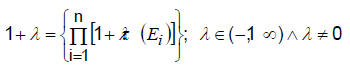(11)

A combination process of cognitive information is presented as: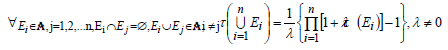(12)

Note that if A = ℜ is a nonempty class such that E, F ∈ ℜ, E ∪ F ∈ ℜ and E – F ∈ ℜ and g satisfies the λ-rule, then τ satisfies the finite λ-rule. The most ideal evaluation, E*, for the quality of the object is the [13,14] and an idea of the Sugeno integral introduced by , where the integral is defined over measurable sets. Let A ∈ A, A is a σ-algebra of sets in ℘(X); f ∈ F, F is the class of all finite non-negative measurable functions defined on measurable space (X, A). Function f: X ⇒ is called a measurable function iff: β is a Borel set and:(13)

Let Fα and Fα+ be called a α-cut and strict α-cut of f, respectively. Instead of the importance measure g, the fuzzy integral of f on A with respect to quantum cognitive measure, τ, is denoted by ƒA f dτ. Sugeno integral is described as:It presents the quantum cognitive evaluation corresponding to both quantitative and qualitative factors in X.
Note that this quantum perception processing problem can be applied also in new model of quantum multiobjective optimization related to other problems such as multiobjective fractional programming, (see Vandana et all.), multiobjective symmetric programming (see R. Dubey et all.) and others. It will be presented in next paper

## Quantum Bit, Qubit, Processing

the traditional informatics, truth is subdivided on separated perceptions, ‘true’ (1) and ‘false’ (0), which are considered as the basic units (bit) of information. In contrast, there are two states in the one, ‘10’, called the quantum bit, qubit, which is considered as the basic units of information in the so called quantum cognitive informatics, see [16-19]. It can be represented by two vectors in the two-dimensional Hilbert space.(16)

which describes the superposition and entanglement of two alternate states: ‘1’ (true) and ‘0’ (false). Mathematically, vector in the Hilbert space using Dirac notation is represented by:

with v = ❘v⟩ we have: v'= [v0 v1 ... vn ]= ⟨v❘

Quantum bits, qubit, is represented by a fixed pair of distinguishable states: ❘0⟩ and ❘1⟩ :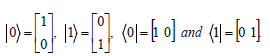(18)

Hilbert space is a vector space with an inner product, for example, numbers 0 and 1 are resulted of inner products: <1|0> and <1|1> respectively: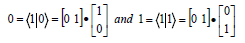(19)

A way of combining vector spaces into larger vector space is called the tensor product, ⊗. Each possible bit configuration in the quantum superposition is denoted by the tensor product of its counterpart cognitive bits. Two columns vectors |u> and |v> of lengths m and n yield a column vector of length m.n when tensored shown in the following equations: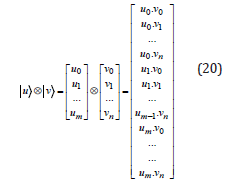(20)

For example, combining 2-vector spaces, |0⟩ and |0⟩ , |1⟩ and |1⟩ and 3-vector spaces, |1⟩ , |0⟩ and |1⟩ ,(21)

### Two qubits processing

On the one hand, classical information processing for two intuitive perceptions: ‘being’ = 1, ‘nonbeing’ = 0, leads to 21

separated intuitive possibilities:
‘being’ or ‘nonbeing’ = {0, 1} = 21 intuitive possibilities (22)
On the other hand, two qubits processing result in a set of 22 quantum possibilities in the informatics form:
‘being-nonbeing’ = {00, 01, 10, 11} = 22 quantum possibilities (23)
Thus, two qubits processing gives us equal result to the result derived from quantum perception processingi (eq. 3) and enables us to create the exponential growth 22 possibilities.
The quantum cognitive superposition ψ of multiple two bit system will be presented as a linear combination of the following basis vectors:Where t0 is the scalar amplitude of quantum cognitive measuring of ❘0 ⟩ t1 is the complex scalar amplitude of quantum cognitive measuring of ❘01 ⟩, …, and t3 the amplitude of quantum cognitive measuring of ❘1 ⟩.

### Three qubits processing

It is represented by a set of 23 possible cognitive possibilities: ‘being-nonbeing’ = {000 001 010 011
100 101 110 111} = 23 quantum possibilities (26)

The use of the tensor products, ⊗, (eq. 20) leads to 23 cognitive quantum states ❘000⟩ , ❘001⟩ , ❘010 ⟩, ❘011⟩ , ❘100⟩ , ❘101 , ❘110⟩ , ❘111⟩ . The superposition ❘ψ⟩ of multiple 3-cognitive bit system is represented as a linear combination of the following basis vectors: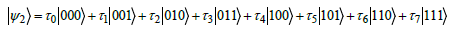(27)

### Four qubits processing

Four qubits processing results in 24 quantum possibilities:
‘being-nonbeing’ = {0000 0001 0010 0100
1000 1001 1010 1100
1110 1101 1011 0110
0011 0101 0111 1111} = 24
quantum possibilities (28)

Thus, four qubits processing gives us the equal result to result derived from quantum perception processing using four quantum possibilities (eq. 4).  The use of the tensor products, Ä, leads to 24 quantum cognitive states: |0000⟩, |0001⟩, ..., |1111⟩. . From that, the cognitive superposition  of multiple 4-cognitive bit system is represented as a linear combination of the following basis vectors: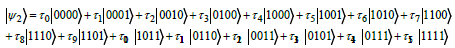(29)

For a cognitive quantum computer, each quantum cognitive bit in the register is in a superposition of |0⟩ and |1⟩ , and thus a register of n qubits is in the superposition of all 2n bit strings that can be expressed in n qubits. A cognitive quantum registers of 3 qubits, for example, using quantum measures t0, t1, ..., t7, should be:(30)

Quantum cognitive logic gates like quantum logic gates maps quantum superposition to another state that allows the evolution of the system to the desired final state. Using the combinations of logic gates - elementary building block of a digital circuit, complex operations can be performed. We should express the XOR gate circuit with cognitive inputs t, t1 and cognitive output Q using IEEE XOR symbol according to distinctive shape (IEEE Std 91/91a- 1991), we have in Figure 3.

The algebraic expression of the XOR gate should be presented as follows.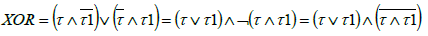(31)

It indicates that quantum perception processing through the human brain and quantum bit processing through quantum computer are based on the same quantum philosophy and quantum logic.

## Conclusion

The Hegel’s idea of quantum perception indicates another capability of the human brain – Handling quantum cognitive processing with the use of quantum perception (Yogaya) allows us to increase the possible profit exponentially up to 2n. Quantum perception originated from the human brain is the consequence of the consciousness process originated from activity of the neural net. It is based on physical and chemical rules of the neural cells, quantum philosophy and quantum logic which are regarded as the foundation of the human thoughts and real time intelligence of the human brain. We would like to exploit the advantages of dynamic quantum cognitive dimension to build a new approach of QI based on a set of the rigorous principles of the quantum philosophy, quantum logic and quantum cognitive information computing using quantum cognitive measurements in order to transcend limits of intuitive perceptions (Laukika) processing towards knowing by quantum perceptions (Alaukika) processing. The characteristic similarity of quantum cognitive processing and quantum bit processing allows us to simulate human thinking processes and create the ability to simulate human brain activity and harness its power by quantum cognitive computations. It helps us to build a new approach of embedded software design, intended to form a key component of the infrastructure of IoT technology, which creates a new model of machine learning and to build a new approach of AI named quantum intelligence QI using human emotion recognition techniques, see [20-22]. The next task should be considering the similarity of the human thinking and the quantum cognitive information processing for the development of quantum cognitive informatics, which allows us to move toward a new architecture of computer that acts as the human brain in the future.

1. Landauer R (1998) Information is inevitably physical, published in Feynman and computation. Taylor and Francis Chapter 8: 16
2. Brouwer LEJ (1975) Collected Works 1 Philosophy and Foundation of Mathematics. A Heyting (Eds.), Amsterdam North-Holland
3. Brouwer LEJ (1976) Collected Works 2 Philosophy and Foundation of Mathematics. A Heyting (Eds.), Amsterdam North-Holland.
4. Heyting A (1965): Intuitionism an Introduction. Philipapers.
5. Łukasiewicz J (1920) O logice trójwartościowej. Ruch Filozoficzny 5: 169-170.
6. Łukasiewicz J, Tarski A (1930) Untersuchungen Uber den Aussagenkalkul, Comptes-rendus des seances de la Societe des Sciences et des Lettres de Varsovie Cl 23: 30-50.
7. Łukasiewicz J (1987) On Aristotle's Contradiction Principle; Library of Contemporary Philosophers; PWN. Warsaw, Poland.
8. Frege FLG (1980) On Sense and Reference by M Black in Translations from the Philosophical Writings of Gottlob Frege, P Geach and M Black (Eds. and trans.), Oxford: Blackwell, (3rd edn) 100: 25-50.
9. Tran C (2017) Artificial Brain and "Human-Machine" Integration. Journal of Computer Science Applications and Information Technology 2(3): 1-7
10. Tran C (2013) Meta-concept and intelligent data processing in the geotechnical fields. Advanced Computational Methods for Knowledge Engineering 479: 169-186.
11. Tran C (2014) Cognitive information processing. J Comput Sci 1(4): 207–218.
12. Tran C (2016) The Internet of Thing and the Brain of Thing, on Research Gate website.
13. Sugeno M (1977) Fuzzy measures and Fuzzy integrals: A survey. Fuzzy Automata and Decision Processes. Amsterdam, North-Holland 2(3): 89-102.
14. Sugeno M, Takagi T (1983) Multi-Dimensional Fuzzy Reasoning. Fuzzy set and System 9(1-3): 313-325.
15. Wang Z, Klir, George (1992) Fuzzy Measure Theory. Plenum Press. N.Y. and London.
16. Robert S Smith, Michael J Curtis, William J Zeng (2016) A Practical Quantum Instruction Set Architecture. Rigetti Computing 2: 1-15.
17. Simon DR (1994) On the Power of Quantum Computation. Proc. 35th Ann Symp Foundations of Computer Science. IEEE Press.
18. Shor PW (1995) Polynomial-Time Algorithms for Prime Factorization and Discrete Logarithms on a Quantum Computer. SIAM Journal on Computing 26(1997): 1484.
19. Shor PW (1995) Scheme for reducing decoherence in quantum computer memory. Phys Rev A52 R2493.
20. Lin YP, Wang CH, Wu TL, Jeng SK, Chen JH (2009) EEG-based emotion recognition in music listening: A comparison of schemes for multiclass support vector machine. In: ICASSP, IEEE International Conference on Acoustics, Speech and Signal Processing - Proceedings, Taipei, pp: 489-
21. Murugappan M, Rizon M, Nagarajan R, Yaacob S, Zunaidi I, et al. (2008) Lifting scheme for human emotion recognition using EEG. In. IEEE.
Close## Online Submission System

### Submit ManuscriptClick here for Manuscript Sample Templates### Drag and drop files here

or

( For multiple files submission, zip them in a single file to submit. For file zipping software Download )